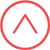Candidates having First Class or 60% marks (55% for SC/ST) in Bachelor's Degree in any Engineering discipline with a valid GATE score in Aerospace Engineering (AE) / Mechanical Engineering (ME) / Electrical Engineering (EE) / Electronics and Communication Engineering (EC) / Instrumentation Engineering (IN) / Chemical Engineering (CH).

Candidates having two years of relevant work experience are exempted from requirement of GATE score, provided their candidature is sponsored by the employer. They are not eligible for award of Teaching (TA) / Research Assistantship (RA).

The written test will be based on the following content:

Control System

Basic control system components; block diagrammatic description, reduction of block diagrams. Open loop and closed loop (feedback) systems and stability analysis of these systems. Signal flow graphs and their use in determining transfer functions of systems; transient and steady state analysis of LTI control systems and frequency response.
Tools and techniques for LTI control system analysis: root loci, Routh-Hurwitz criterion, Bode and Nyquist plots.
Control system compensators: elements of lead and lag compensation, elements of Proportional-IntegralDerivative (PID) control. State variable representation and solution of state equation of LTI control systems.

Engineering Mathematics

Linear Algebra: Matrix Algebra, Systems of linear equations, Eigen values and eigen vectors.
Calculus: Mean value theorems, Theorems of integral calculus, Evaluation of definite and improper integrals, Partial Derivatives, Maxima and minima, Multiple integrals, Fourier series. Vector identities, Directional derivatives, Line, Surface and Volume integrals, Stokes, Gauss and Green’s theorems.
Differential equations: First order equation (linear and nonlinear), Higher order linear differential equations with constant coefficients, Method of variation of parameters, Cauchy’s and Euler’s equations, Initial and boundary value problems, Partial Differential Equations and variable separable method.
Complex variables: Analytic functions, Cauchy’s integral theorem and integral formula, Taylor’s and Laurent’ series, Residue theorem, solution integrals.
Probability and Statistics: Sampling theorems, Conditional probability, Mean, median, mode and standard deviation, Random variables, Discrete and continuous distributions, Poisson,Normal and Binomial distribution, Correlation and regression analysis.
Numerical Methods: Solutions of non-linear algebraic equations, single and multi-step methods for differential equations.
Transform Theory: Fourier transform, Laplace transform, Z-transform.Website Maintained by Webteam - SysCon